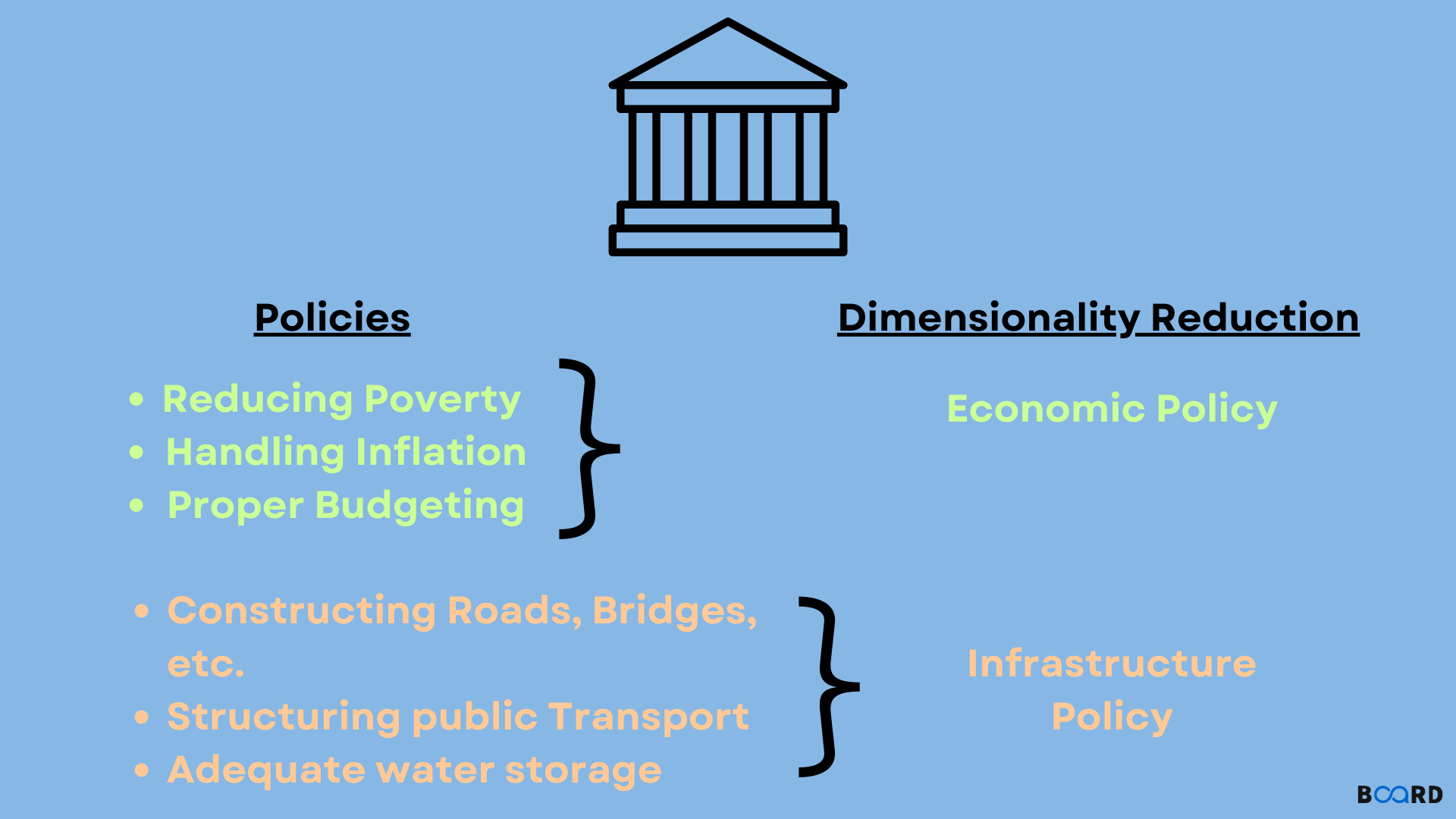# Dimensionality Reduction in Machine Learning## Overview

In classification problems of machine learning sometimes there exist several factors known as features that affect the final classification. The more features, the more difficulty occurs in visualizing and operating upon the training dataset.

Seldom, there is a correlation between many of the features and therefore they become redundant. Then comes in the scene the concept of dimensionality reduction. It is the method of decreasing the count of considered random variables by finding a set of principal variables.

## Scope

• What is dimensionality reduction?
• Components of dimensionality reduction.
• Methods of dimensionality reduction.

• ML algorithms.
• Other unrelated ML concepts.

## What is dimensionality reduction?

Dimensionality is the number of columns, variables, or input features in a given dataset. Dimensionality reduction is the technique of decreasing these features. A dataset may contain a large count of input features in several cases, complicating the task of predictive modeling. Since it is hard predicting or visualizing the training dataset with a huge count of features, dimensionality reduction is required.

Dimensionality reduction techniques are a method of transforming a dataset with high dimensions into one with fewer dimensions, while also making sure that it still provides similar information. Dimensionality reduction is widely used in machine learning for finding a better-fit predictive model during solving regression and classification problems.

## Components of dimensionality reduction

Dimensionality reduction has the following two components:

### Feature selection

In feature selection, we attempt to hunt a subset of the original features (variables) to obtain a tinier subset that can be used in modeling the problem. This can be achieved in one of the following ways:
1. Filter
2. Wrapper
3. Embedded

### Feature extraction

Feature extraction decreases the amount of data in a space with a high number of dimensions to a lower dimension space. Following are a few common techniques:

• Principal component analysis.
• Linear discriminant analysis.
• Kernel PCA.

## Dimensionality reduction methods

The following are some of the prominent dimensionality reduction techniques:

• Auto-Encoder.
• Factor Analysis.
• Score comparison.
• Random Forest.
• Missing Value Ratio.
• Low Variance Filter.
• High Correlation Filter.
• Forward Selection.
• Backward Elimination.
• Principal Component Analysis.

## Conclusion

• The more features, the more difficulty occurs in visualizing and operating upon the training dataset.
• Dimensionality reduction is the method of decreasing the count of considered random variables by finding a set of principal variables.
• Dimensionality is the number of columns, variables, or input features in a given dataset.
• Dimensionality reduction has the following two components: feature selection and feature extraction.